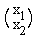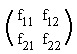# Multiplication of a Vector by a Matrix

Consider a real two-dimensional space R2 which is the space of all ordered pairs of real numbers. (The pairs are ordered in the sense that, say, (3,5)≠(5,3).)

In R2, we are interested in linear transformations f: R2→R2:

(1) f(r x + s y) = r f(x) + s f(y)

where r and s a (real) scalars and x,y∈R2. While working on such transformations the English mathematician Arthur Cayley (1821-1895) devised in 1857 matrix algebra.

From the definitions of vector addition and componentwise multiplication by a scalar,

(2) x = (x1,x2) = x1(1,0) + x2(0,1)

Apply now (1) to (2): f(x) = x1f(1,0) + x2f(0,1), where f(1,0) that should more correctly be written as f((1,0)), is the result of applying f to the vector (1,0). A similar remark holds for f(0,1) and f(x1,x2) in the following. Let f(1,0)=(f11,f21) and f(0,1)=(f12,f22). Then

(3) f(x) = f(x1, x2) = x1(f11, f21) + x2(f12, f22) = (x1f11 + x2f12, x1f21 + x2f22)

Assume g is another linear transformation and g(1,0)=(g11, g21) and g(0,1)=(g12, g22). Then by (3),

(4) g(f(x)) = ((x1f11 + x2f12)g11 + (x1f21 + x2f22)g12, (x1f11 + x2f12)g12 + (x1f21 + x2f22)g22)

or, after regrouping,

(4') g(f(x)) = (x1(f11g11 + f21g12) + x2(f12g11 + f22g12), x1(f11g12 + f21g22) + x2(f12g12 + f22g22))

We see that a composition g(f(x)) of two linear transformations is in turn linear. Furthermore,

(5) g(f(1,0)) = (f11g11 + f21g12, f11g21 + f21g22), and
g(f(0,1)) = (f12g11 + f22g12, f12g21 + f22g22)

Now, a pair of numbers x1,x2 might be written as either a vertical (2x1 matrix) or a horizontal (1x2 matrix) vector. Given the limitations of HTML, the horizontal convention is a real life saver and has been used so far. However, I must note that the vertical notations are, by far, more common. Depending on the notations (3) and (5) may be rewritten variously in a vector-matrix format:

 x (x1, x2)Ff(x) xF Fx g(f(x)) xFG GFx

What we arrived at is that a linear transformation of a vector space may be expressed as a product of a matrix and a vector. Composition of two linear transformations is represented by a product of the corresponding matrices. The claim is more general than what was actually shown. R2 is known as an arithmetic vector space. The set of all combinations rsin(x) + scos(x), where x changes over some interval, is another example of a 2-dimensional vector space whose elements look differently from those of R2. However, as we already remarked, vector spaces of the same dimensionality are isomorphic, and one way to establish a correspondence (isomorphism) between them is by selecting bases and identifying their vectors with tuples of coordinates. For example, a vector rsin(x) + scos(x) could be identified with an ordered pair (r,s). Under this correspondence, sin(x) and cos(x) appear as (1,0) and (0,1), respectively.

To sum up, selecting bases in vector spaces leads to identification of vectors with tuples of coordinates (horizontal or vertical). Horizontal tuples are usually called row vectors whereas the vertical ones are known as column vectors. Matrices turn up as representing linear transformations for a fixed pair of bases. To obtain the result of applying a transformation to a vector multiply the corresponding matrix and the tuple. The product of two matrices represents the composition (product) of two transformations (functions.)

## Reference

1. H.Eves, Great Moments in Mathematics After 1650, MAA, 1983### What Can Be Multiplied?